Courses

# Test: Geometrical Isomerism- 2

## 30 Questions MCQ Test Chemistry for JEE | Test: Geometrical Isomerism- 2

Description
This mock test of Test: Geometrical Isomerism- 2 for JEE helps you for every JEE entrance exam. This contains 30 Multiple Choice Questions for JEE Test: Geometrical Isomerism- 2 (mcq) to study with solutions a complete question bank. The solved questions answers in this Test: Geometrical Isomerism- 2 quiz give you a good mix of easy questions and tough questions. JEE students definitely take this Test: Geometrical Isomerism- 2 exercise for a better result in the exam. You can find other Test: Geometrical Isomerism- 2 extra questions, long questions & short questions for JEE on EduRev as well by searching above.
QUESTION: 1

Solution:
QUESTION: 2

### Which compound below has four stereoisomers ?

Solution:

Since in compound a, b and d we have symmetry in the molecule, so both double bonds are identical. THerefore, stereoisomerism is counted from only one bond. This will give us 2 isomers.
In compound c, both double bonds are different, so both will involve GI. N has a lone pair which will also be counted. So, we have 4 isomers in all.

QUESTION: 3

### Which compound below is capable of showing geometrical isomerism ?

Solution:

The correct answer is option B
CH3 – CH = C = C = CH – CH3 will show geometrical isomerism because it is odd cumulene (3 pie bond) and odd cumulene always shows geometrical isomerism

QUESTION: 4

consider the following reaction.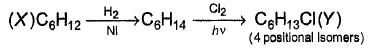How many different isomers of X satisfy the above condition ?

Solution:

The correct answer is Option C.
If C6H3Cl has 4 positional isomers then it’s structure can be;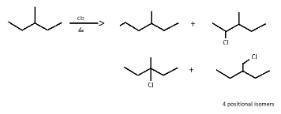So, X can be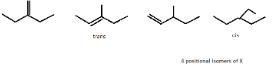QUESTION: 5

Which isomer below has a stable intramolecular H-bond?

Solution:
QUESTION: 6

A ketone with molar mass of 100 g, has only one positional isomer. How many different enol isomers exist for this ketone?

Solution:
QUESTION: 7

Carbonyl compound condenses with ammonia as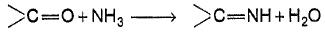How many diffrent dilimines would be obtained on condensation of 2,4- pentanedlone with excess of ammonia ?

Solution:
QUESTION: 8

How many cyclic Isomers (structural and geometrical only) exist for C5H10

Solution:

5 isomers are possible. C5H10(CnH2n). Molecules having the CnH2n  formula are most likely to be cyclic alkanes. The five isomers are: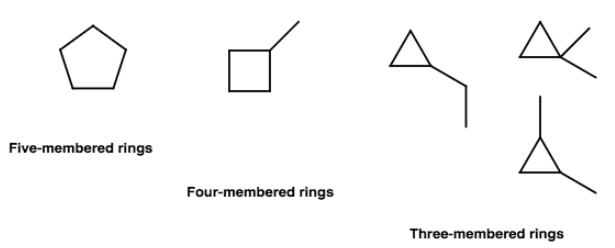QUESTION: 9

How many geometrical isomers exist for the molecule shown below ?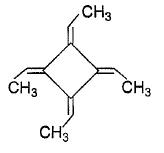Solution:

The correct answer is option B

Threegeometrical isomers exits of the molecule given cis-cis, tran-trans and cis-trans.

QUESTION: 10

If chlorocyclohexane is subjected to further chlorination, how many different isomers (geometrical plus structural only) of dichiorocyclohexane would be produced?

Solution:

The correct answer is option D
There will be three constitutional isomers i.e. 1,2 dichloro cyclohexane,1,3 dichloro cyclohexane and1,4 dichloro cyclohexane.Each constitutional isomer will have cis and trans configuration thereby making six isomers in all.Also there will be two optically active isomers.
You can understand it better through these infographics
1,2 dichloro cyclohexane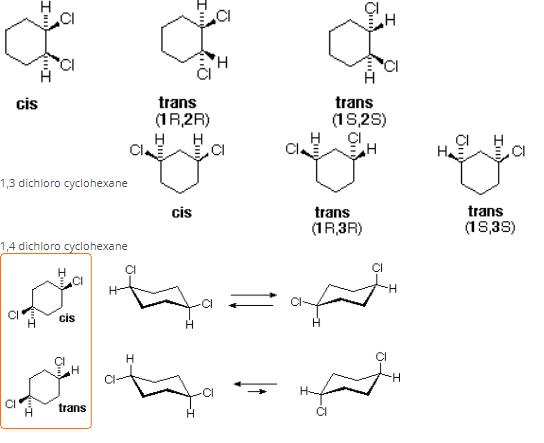*Multiple options can be correct
QUESTION: 11

Direction (Q. Nos. 11-18) This section contains 8 multiple choice questions. Each question has four
choices (a), (b), (c) and (d), out of which ONE or  MORE THANT ONE  is correct.

Q.

Choose the correct statement(s) regarding isomers of C2H2C12.

Solution:

The correct answers are Option B,C and D.
C2H2C12 => 3 isomers possible.

1.  1,2-dichloroethane ---> cis
2.  1,1-dichloroethane ---> trans

(1)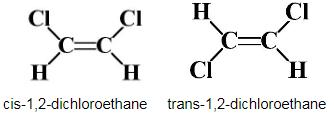Net dipole moment=0
So, no polar isomer.
(2)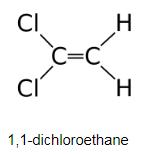*Multiple options can be correct
QUESTION: 12

Dibromo derivative of a hydrocarbon has molar mass of 200 g (Br = 80) and none of the isomers have any ring structure. Which is/are the correct deduction regarding isomers?

Solution:

The correct answers are option B & C.
Compound is dibromoethene, it can have 3 isomers (two geometrical isomers (cis & trans) of 1,2 dibromoethene and one is 1,1 dibromoethene).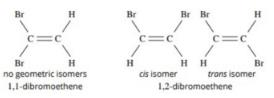*Multiple options can be correct
QUESTION: 13

Which of the following carbonyls have four enol isomers?

Solution:

The correct answers are Options C and D.

Enolization involves the α-carbon atom. The ketone 2,4-dimethyl-3-pentanone gives a single enol, since the two α carbons are equivalent. An enol is not a resonance form of a carbonyl compound; the two are constitutional isomers of each other.

*Multiple options can be correct
QUESTION: 14

Pick up the correct statements regarding isomers of C2FCIBrt

Solution:
*Multiple options can be correct
QUESTION: 15

Enol isomer of which of the following forms six membered stable ring through intramolecular H-bonding?

Solution:
*Multiple options can be correct
QUESTION: 16

Which is/are true regarding pair of geometrical isomers?

Solution:

a) Geometric isomerism occurs when two structures with the same connectivity are not inconvertible.
b) The boiling points of the isomers might be different enough that you can separate them by fractional distillation. The affinities for the stationary phase may be different enough that they can be separated by gas chromatography.
c) Geometric isomers are diastereomers, i.e. they are stereoisomers that are not enantiomers. The prefixes cis and trans refer to the relative dispositions of the substituents attached to the doubly bonded carbon atoms. In the cis diastereomer the hydrogen atom attached to one carbon is on the same side of the double bond as the hydrogen attached to the other carbon. In the trans stereoisomer they are on opposite sides.

*Multiple options can be correct
QUESTION: 17

Which of the following compounds have more than one pairs of stereoisomers?

Solution:
*Multiple options can be correct
QUESTION: 18

A hydrocarbon X (M = 140 g/mol) on catalytic hydrogenation gives C10H22 (Y). Y on chlorination gives only two positional isomers with molecular formula C10H21C1. Which is/are correct about X and Y?

Solution:
QUESTION: 19

Direction (Q. Nos. 19 and 20) Choice the correct combination of elements and column I and coloumn II  are given as option (a), (b), (c) and (d), out of which ONE option is correct.

Q.

Match the quantity from Column I with the types of isomerism from Column II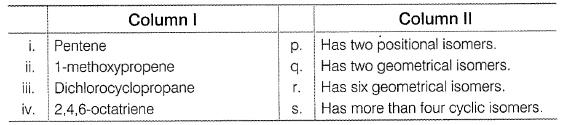Codes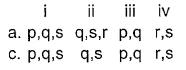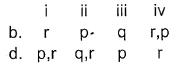Solution:
QUESTION: 20

Match the systems in Column I with their corresponding characterstics from Column II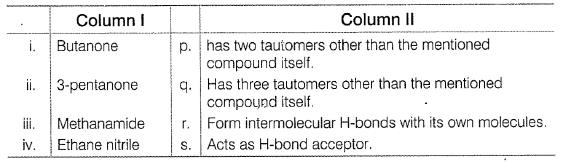Codes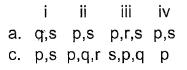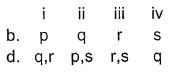Solution:

The correct answer is option A
i. Butanone- q) Has three tautomers other than the mentioned compound.
s) Acts as H-bonds acceptor.
ii. 3-pentanone- p) has two tautomer’s other than the mentioned compound itself
s) Acts as H-bonds acceptor.
iii. Methanamide - p) has two tautomer’s other than the mentioned compound itself
r) Form intermolecular H-bonds with its own molecules.
s) Acts as H-bonds acceptor.
Iv. Ethane nitrite - p) has two tautomer’s other than the mentioned compound itself
s) Acts as H-bonds acceptor.

QUESTION: 21

Direction (Q. Nos. 21-26) This section contains 2 paragraph, wach describing  theory, experiments, data etc. three Questions related to paragraph have been  given.Each question have only one correct answer among the four given  ptions  (a),(b),(c),(d)

Passage I

A number of unsaturated hydrocarbons have the same molecular formula C11H22. All of these hydrocarbons on catalytic hydrogenation gives the same 3,4,6-trimethyloctane.

Q.

How many structural isomers of the starting hydrocarbon, on catalytic hydrogenation can give the mentioned alkane?

Solution:
QUESTION: 22

Passage I

A number of unsaturated hydrocarbons have the same molecular formula C11H22. All of these hydrocarbons on catalytic hydrogenation gives the same 3,4,6-trimethyloctane.
Q.
How many of the above unsaturated hydrocarbons are capable of exhibiting geometrical isomerism?

Solution:
QUESTION: 23

Passage I

A number of unsaturated hydrocarbons have the same molecular formula C11H22. All of these hydrocarbons on catalytic hydrogenation gives the same 3,4,6-trimethyloctane.
Q.

If the product alkane is 3,6-dimethyloctane, how many different isomers. (structural plus geometrical only) of alkenes can give this product?

Solution:
QUESTION: 24

Passage II

Consider the dollowing molecules to answer the next three questions.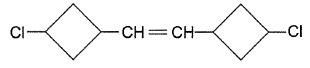Q.

How many stereoisomers exist for this molecule ?

Solution:

the number of stereogenic centres which comprises of optically active carbon and the carbon showing geometrical isomerism. In the above picture, there is only one geometric isomer and chiral carbon. So there is 2 stereogenic centre.
stereoisomers = 2n
=22
= 4

QUESTION: 25

Passage II

Consier the following molecules to answer the next three questions.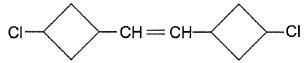Q.

Treatment of halogenated hydrocarbon with NaBH4 reduces secondary and tertiary halides but olefinic bonds are not affected. If the above mentioned compound is reduced with NaBH4, how many different isomers would be produced?

Solution:
QUESTION: 26

Passage II

Consider the following molecules to answer the next three questions.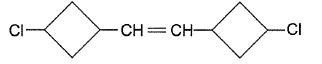Q.

If the given compound is carefully reduced so that only double bond is reduced, leaving halide bonds intact, how many different stereoisomers would be produced?

Solution:
*Answer can only contain numeric values
QUESTION: 27

Direction (Q. Nos. 27-30) This section contains 4 questions. when worked out will result in an integer from 0 to 9 (both inclusive)

Q.

For hydrocarbon with molecular formula C5H8, how many acyclic isomers without consecutive pi-bonds are possible?

Solution: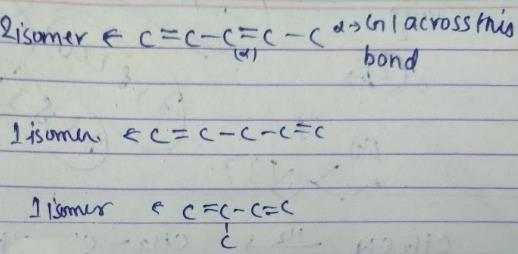As we are restricted to have molecules without consecutive double bond, we will have 4 structure.
So, according to me three would be 4 isomers possible .

*Answer can only contain numeric values
QUESTION: 28

How many different isomers of alkene with molecular formula C7H14, on catalytic hydrogenation, can give 3-methyl hexane?

Solution: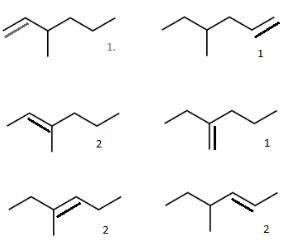*Answer can only contain numeric values
QUESTION: 29

How many geometrical isomers exist in 1,2,4-trichlorocyclopentane?

Solution:
*Answer can only contain numeric values
QUESTION: 30

How many geometrical isomers exist for 1-bromo-2-chloro-3-fluorocyclopropane?

Solution: